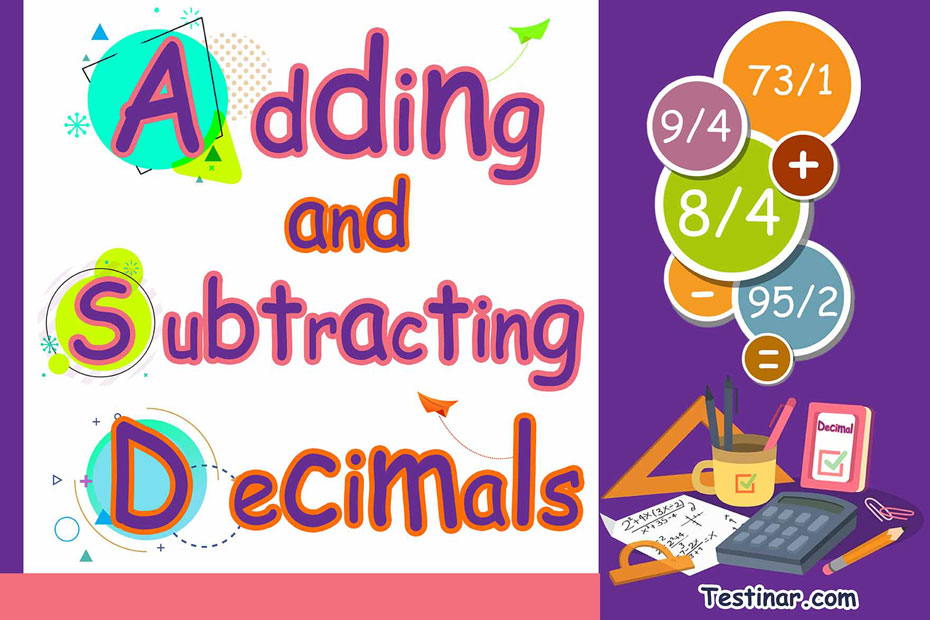## How to Add or Subtract Decimals

In mathematics, a decimal can be defined as a number which has two parts: a whole part and a fractional part, and these two parts are separated by a decimal point. The whole part always represents a number greater than one, while the fractional part, i.e., the part after the decimal, always represents a number less than one.

For example, let’s take the number $$15.74$$. Now here the whole part is represented by $$15$$, whereas the fractional part $$(\frac{74}{100} \ )$$ is represented by $$74$$. Here $$74$$ can also be denoted as the decimal part, as it lies after the decimal point.

#### Terminating and Non-Terminating Decimals

Terminating decimals are the one which have an end whereas non-terminating decimals don’t have an end. They are simply recurring digits that go on and on.

For example, $$3.333333$$ , $$( \frac{10}{3} \ )$$ is a recurring/non-terminating decimal, whereas $$2.50$$ , $$(\frac{10}{4} \ )$$ is a terminating decimal.

#### The Concept of Preceding Powers of 10

Now, there’s a very interesting concept linked to decimal numbers. This concept is known as the preceding powers of $$10$$. All decimal numbers are based on this concept. So, as we move from left to right in a decimal number, basically, the place value of every digit gets divided by $$10$$. So, the first digit after a decimal could be represented as $$\frac{1}{10}$$ , second as $$\frac{1}{100}$$ and so on.
So, from this concept, we can easily find out the expanded form of a decimal.

For example, let’s take the decimal number $$16.457$$. The expanded form could be written as: $$10 + 6 + \frac{4}{10} + \frac{5}{100} + \frac{7}{1000}$$
Also, $$16.457$$ can be represented as $$16 \frac{457}{1000}$$ in mixed fraction terms.

#### How to Add or Subtract 2 Decimals

To add or subtract 2 decimals, use the following steps:

• First, line up the two numbers as you would do in simple addition or subtraction.
• Next add zeroes to the right of decimals to match number of digits (if not same). For example, when performing $$(2.5-2.25)$$, convert $$2.5$$ to $$2.50$$.
• Then perform simple column addition or subtraction.

### Exercises for Adding and Subtracting Decimals

1) \cfrac{-\begin{align}114.334\\62.120\end{align}}{}

2) \cfrac{-\begin{align}129.743\\34.230\end{align}}{}

3) \cfrac{+\begin{align}121.373\\31.220\end{align}}{}

4) \cfrac{+\begin{align}114.648\\31.330\end{align}}{}

5) \cfrac{+\begin{align}129.494\\56.750\end{align}}{}

6) \cfrac{+\begin{align}136.978\\42.880\end{align}}{}

7) \cfrac{-\begin{align}136.712\\16.530\end{align}}{}

8) $$123.428 \ + \ \color{red}{\underline{}\underline{}\underline{}} \ = 139.198$$

9) $$130.666 \ + \ \color{red}{\underline{}\underline{}\underline{}} \ = 205.996$$

10) $$123.389 \ + \ \color{red}{\underline{}\underline{}\underline{}} \ = 173.919$$

1) \cfrac{-\begin{align}114.334\\62.120\end{align}}{\color{red}{\quad52.214}}
First line up the numbers:\cfrac{-\begin{align}114.334\\62.120\end{align}}{} , Start with the thousandth place  $$4 - 0 = 4$$, so \cfrac{-\begin{align}114.334\\62.120\end{align}}{ 0.004} . Continue with hundredth place. $$3-2=1$$ and then the tenth place $$3-1=2$$ . \cfrac{-\begin{align}114.334\\62.120\end{align}}{0.214} . Finally subtract the integer parts $$114 - 62 = 52$$,   \cfrac{-\begin{align}114.334\\62.120\end{align}}{52.214}.
2) \cfrac{-\begin{align}129.743\\34.230\end{align}}{\color{red}{\quad95.513}}
First line up the numbers:\cfrac{-\begin{align}129.743\\34.230\end{align}}{} , Start with the thousandth place  $$3 - 0 = 3$$, so \cfrac{-\begin{align}129.223\\34.730\end{align}}{ 0.003} . Continue with hundredth place. $$4-3=1$$ and then the tenth place $$7 -2 =5$$ . \cfrac{-\begin{align}114.334\\62.120\end{align}}{0.513} . Finally subtract the integer parts $$129 -34 = 85$$,   \cfrac{-\begin{align}129.743\\34.230\end{align}}{95.513}.
3) \cfrac{+\begin{align}121.373\\31.220\end{align}}{\color{red}{\quad152.593}}
First line up the numbers:\cfrac{+\begin{align}121.373\\31.220\end{align}}{} , Start with the thousandth place  $$3 + 0 = 3$$, so \cfrac{+\begin{align}121.373\\31.220\end{align}}{0.003} . Continue with hundredth place $$7+2=9$$ and then the tenth place $$3+2=5$$ . \cfrac{+\begin{align}121.373\\31.220\end{align}}{0.593} . Finally subtract the integer parts $$121+31 = 152$$,  \cfrac{+\begin{align}121.373\\31.220\end{align}}{152.593}.
4) \cfrac{+\begin{align}114.648\\31.330\end{align}}{\color{red}{\quad145.978}}
First line up the numbers:\cfrac{+\begin{align}114.648\\31.330\end{align}}{} , Start with the thousandth place  $$8+ 0 = 8$$, so \cfrac{+\begin{align}114.648\\31.330\end{align}}{0.008} . Continue with hundredth place $$4+3=7$$ and then the tenth place $$6+3=9$$ . \cfrac{+\begin{align}114.648\\31.330\end{align}}{0.978} . Finally subtract the integer parts $$114+31 = 145$$,  \cfrac{+\begin{align}114.648\\31.330\end{align}}{145.978}.
5) \cfrac{+\begin{align}129.494\\56.750\end{align}}{\color{red}{\quad186.244}}

6) \cfrac{+\begin{align}136.978\\42.880\end{align}}{\color{red}{\quad179.858}}

7) \cfrac{-\begin{align}136.712\\16.530\end{align}}{\color{red}{\quad120.182}}

8) $$123.428 \ + \ \color{red}{\underline{}\underline{}\underline{}} \ = 139.198 \ \ \Rightarrow 139.198 \ - \ 123.428 \ = \ \color{red}{\underline{}\underline{}\underline{}}$$
\Rightarrow \cfrac{-\begin{align}139.198\\123.428\end{align}}{\color{red}{\quad15.770}}

9) $$130.666 \ + \ \color{red}{\underline{}\underline{}\underline{}} \ = 205.996 \ \ \Rightarrow 205.996 \ - \ 130.666 \ = \ \color{red}{\underline{}\underline{}\underline{}}$$
\Rightarrow \cfrac{-\begin{align}205.996\\130.666\end{align}}{\color{red}{\quad75.330}}

10) $$123.389 \ + \ \color{red}{\underline{}\underline{}\underline{}} \ = 173.919 \ \ \Rightarrow 173.919 \ - \ 123.389 \ = \ \color{red}{\underline{}\underline{}\underline{}}$$
\Rightarrow\cfrac{-\begin{align}173.919\\123.389\end{align}}{\color{red}{\quad50.530}}

## Adding and Subtracting Decimals Quiz

### FSA Grade 6 Math Prep 2020

$19.99$14.99

### GED Math Full Study Guide 2022-2023

$25.99$13.99

### STAAR Grade 8 Mathematics Formulas

$6.99$5.99

### FTCE Math Study Guide 2020-2021

$20.99$14.99